# TUTORIAL ON SUPERCUBE SOLVING (1) (Basic Notions)

This series of webpages provide a tutorial on how to solve Supercubes - a special type of Rubik's cubes. But before providing a detailed guide on the solution process, I must first introduce the basic concepts of a Supercube, the notation I am going to use, and some useful facts about Supercubes. This is the purpose of this introductory webpage. Readers who wish to play Supercubes online may visit the Fun with Rubik's Cube webpage (choose "Virtual Cubes" and then a specific size (2 × 2, 3 × 3, etc.) of cubes and then "Picture Cubes"). Please also note that most figures of Supercubes on this series of webpages are captured from the abovementioned website.

## What ia a Supercube?

By "Supercube" (also called "picture cube") I mean a Rubik's cube each facelet of which has a different asymmetric picture or symbol. Here the term "facelets" refers to the small square areas covering the faces of the cube. For example, each of the 6 faces of a 3 × 3 × 3 Rubik's Cube is covered by 9 facelets. According to the characteristics of the picture / symbol on each facelet, Supercubes can be classified into different types. The two most important types of Supercubes are shown below: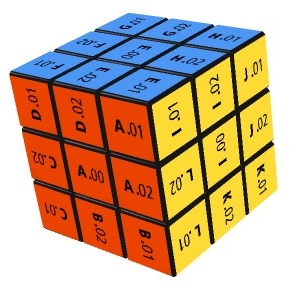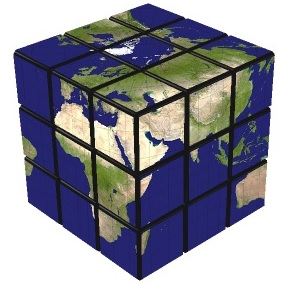The Supercube in the left figure above is not very different from a Rubik's Cube in that the facelets on each face all have the same color, except that a distinguishing symbol is added onto each facelet. Each of these distinguishing symbols is made up of an alphabet and a numeral. The numeral is the reference number of the "orbit" which the facelet belongs to. Orbit is in fact a mathematical term. Roughly speaking, two facelets at different positions of the cube belong to the same oribt if you can move one to the other. For example, the 24 facelets of the corner pieces (a cube has 8 corner pieces each having 3 facelets) all belong to the same orbit (numbered "01" in the figure above) because they can be moved to each other's positions. The alphabet on each facelet is to distinguish different facelets on the same orbit. For example, since there are 24 facelets on the "01" orbit, 24 alphabets are used to label these facelets, i.e. "A.01", "B.01", "C.01", "D.01", etc. In contrast, the Supercube in the right figure above differs from a Rubik's Cube drastically in that this Supercube does not have distinctive color for each face. Moreover, each facelet has a complicated picture showing a part of the Earth. For convenience, in what follows I will refer to the above two Supercubes as the "Orbit Cube" and "Earh Cube", respectively.

Intuitively, it seems that the Earth Cube is harder to solve than the Orbit Cube because each of the six faces of the Earth Cube has the same background color and one cannot determine which corner / edge pieces belong to which faces according to the color. This is indeed true for a novice player, who has to memorize the picture of each facelet. For example, a player of the Earth Cube has to recall that the corner piece made up of the following facelets should be located at the front-right-top corner of the cube, with each of the following three facelets facing forward, rightward and upward, respectively: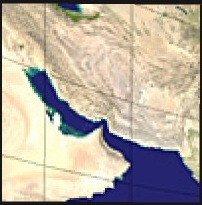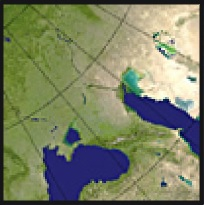However, apart from this complication, the solution procedure of the Earth Cube is in fact no different from that of the Orbit Cube. Both types of cubes are of the same nature and they are just different versions of Supercubes. For this reason, in the following discussion I will use the Orbit Cube as a typical example of Supercubes.

## Components of a Supercube

Supercubes come in different sizes, which are commonly denoted by "n × n × n" (or just "n × n", where n is an integer greater than 1). Altneratively, we can also denote the size of a Supercube by its "order". Thus, an nth-order Supercube means the same as an n × n × n Supercube. In both types of notation, the integer n represents the number of layers that a Supercube contains, both vertically and horizontally. For convenience, I classify the layers of a Supercube into two types: the outer surfaces, i.e. the two outermost layers and the inner layers, which can be further classified into two subtypes: the core inner layers, i.e. the very middle layer and the non-core inner layers, i.e. the remaining layers of a Supercube. The following figure illustrates these notions: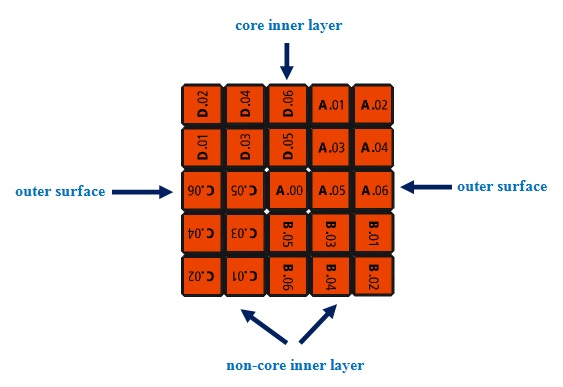Apart from the abovementioned large components, a Supercube also contains smaller components called "pieces". Each piece comprises one to three facelets. The following figure shows the names and examples of the various types of pieces on a 7th-order Supercube:First, we have those pieces each comprising 3 facelets. They are located at the corners of the Supercube and are thus called corner pieces. Next, we have those pieces each comprising 2 facelets. They are located on the edges of the Supercube and are thus collectively called the edge pieces. Depending on their locations on the edges, the edge pieces are classified into two subtypes. The core edge pieces are located at the middle of each edge whereas the non-core edge pieces are located at the other positions of the edges. Finally, we have those pieces each comprising just 1 facelet. They are located in the interior of the faces of the Supercube and are collectively called the center pieces. Depending on their locations in the interior of the faces, the center pieces are classified into four subtypes, which I will introduce in detail in the following paragraphs.

The core center pieces are located at the very center of each face. All other subtypes of center pieces will be collectively called non-core center pieces. These include the point pieces, cross pieces and paired pieces. The point pieces are located at the corners of the internal squares on each face. In the figure above, the pieces labelled "A.05", "B.05", "C.05" and "D.05" are point pieces at the four corners of an internal square on the red face. Please note that the pieces labelled "A.07", "B.07", "C.07" and "D.07" are also point pieces as they are located at the four corners of a smaller internal square on the red face. The cross pieces are located on the internal "cross" on each face (excluding the core center piece). In the figure above, the pieces labelled "A.11", "B.11", "C.11", "D.11", "A.10", "B.10", "C.10", "D.10" are cross pieces. They are all located on the internal cross on the red face.

The paired pieces refer to the remaining center pieces. One characteristic of the paired pieces is that the orbits of these pieces match in pairs in a way such that on each non-core inner layer, the matching pairs either do not appear or both appear at symmetric positions. In the figure above, the "04" and "08" orbits form a pair. For example, one can see that the "D.04" and "C.08" pieces appear at symmetric positions on the same vertical inner layer and the "D.04" and "A.08" pieces also appear at symmetric positions on the same horizontal inner layer.

To understand better the characteristics of paired pieces, we need to look at a larger sized Supercube. Since it is difficult to find pictures of such Supercubes, I draw the following figure which represeents a face of an 11th-order Supercube (the shaded region below represents the core inner layer of the Supercube):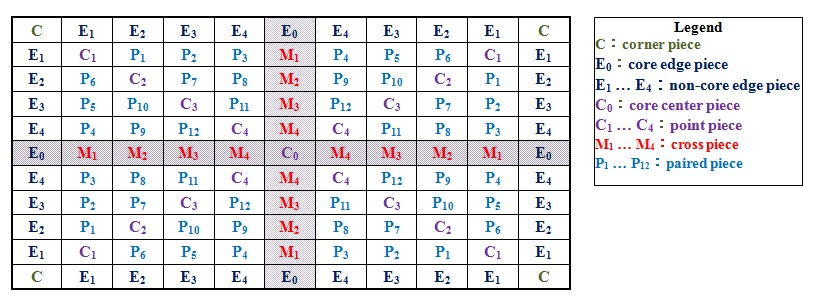In the figure above, the "alphabet + numeral" combinations represent the orbits of the components. We focus on the paired pieces, which are labelled P1 - P12. In this picture, there are 6 matching pairs: P1-P6, P2-P5, P3-P4, P7-P10, P8-P9 and P11-P12. One can clearly see that these matching pairs do have the characteristics of paired pieces described above. For example, the P1 and P6 pieces appear at symmetric positions on the two non-core inner layers containing the cross pieces M1 and M2, and they both do not appear on the two remaining non-core inner layers.

We also need two collective terms for Supercubes of the 4th-order or above: edges and centers. An edge refers to the collection of all adjoining edge pieces, including the core edge piece and the non-core edge pieces, on an edge of the Supercube. Similarly, a center refers to all adjoining center pieces, including the core center piece as well as the point pieces, cross pieces and paired pieces, in the interior of a face of the Supercube. Thus, regardless of the size, every Supercube has 12 edges and 6 centers (plus 8 corners), although Supercubes of different sizes may have different numbers of edge pieces and center pieces.

Finally, please note that not every type of components introduced above exists in all Supercubes. Specifically, the core inner layer and all those pieces located on this layer, including the core edge pieces, core center pieces and cross pieces, only exist in Supercubes of an odd-order (i.e. 3rd-order, 5th-order, 7th-order, etc). The non-core inner layers and all those pieces located on these layers, including the non-core edge pieces and non-core center pieces, only exist in Supercubes of the 4th-order or above. Moreover, the paired pieces only exist in Supercubes of the 6th-order or above.

## Rotations of a Supercube

Since rotations can be done on various layers of a Supercube at various angles and directions, we need a notation to specify clearly all possible rotations. I first discuss the outer surface rotations. I use the six capital letters F, B, R, L, U and D to represent a 90° clockwise rotation of the front, back, right, left, top and bottom outer surface rotations, respectively, and use F−1, B−1, R−1, L−1, U−1 and D−1 to represent the corresponding anticlockwise rotations. The following figure illustrates the 12 outer surface rotations: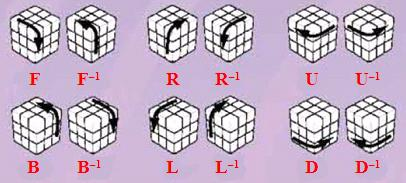I next turn to inner layer rotations. These will be denoted by a capital letter (F, B, R, L, U or D) representing a face plus a subscripted number n representing the nth inner layer counted from that face. Thus, U1−1 represents a 90° anticlockwise rotation of the 1st inner layer counted from the top face. This rotation is illustrated by the following figure: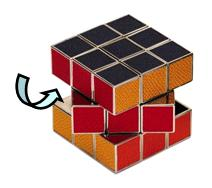Please note that since an inner layer can be counted from either one of the opposite faces, any inner layer rotation can be denoted in two different ways. For example, the rotation shown in the figure above can also be denoted as D1. Moreover, for convenience, sometimes I will also use the subcripted letter m to represent the core inner layer (of an odd-order Supercube). Thus, the rotation shown above can also be denoted as Um−1 or Dm.

Rotations can be composed, i.e. they can be done one after the other. I will denote composed rotations by placing their symbols one after the other, with the leftmost symbol representing the first rotation to be done. Thus, FR2U−1 represents doing an F rotation first, to be followed by an R2 rotation, and then a U−1 rotation.

To represent repetitive rotations succinctly, I will make use of the exponential notation. Thus, instead of writing UU, I will write U2 to represent a 180° clockwise rotation of the top outer surface. The exponential notation can also be used to represent repetitive series of rotations. Thus, (FR2U−1)2 means FR2U−1FR2U−1. Borrowing a law of indicies from mathematics, i.e. x−n = (x−1)n, I will use a negative exponent to represent repetitive anticlockwise rotations. Thus, U−2 means (U−1)2, or equivalently, U−1U−1, i.e. 180° anticlockwise rotation of the top outer surface.

Finally, very often we have to rotate the Supercube as a whole. These will be called whole cube rotations. Since this kind of rotations will not change the relative positions of the Supercube components, it is assumed that they can be freely used and no special notation will be used to represent them.

## Some Useful Formulas

In this section, I introduce some useful formulas for solving Supercubes. The following introduction does not include those formualas that are only used for solving the 3rd-order Rubik's Cube since I assume that the readers already know how to solve this kind of cube. If necessary, please consult 7 Steps Solution Guide.

The first two formulas are called "(XYZ) Formulas". They are so called because they can be used to move a center piece at position X to position Y, the center piece at position Y to position Z, and the center piece at position Z to position X. In mathematics (more precisely Group Theory), this circular transformation is denoted (XYZ). To correctly use these formulas, X must be the position of a center piece on the front face, Y must be the corresponding position on the right face and Z must be a position on the front face reachable from X by a 90° clockwise or anticlockwise rotation of the front face, subject to a use condition, i.e. positions X and Z must not lie on the same horizontal line. The (XYZ) formulas come in two forms. If Z is the position reachable from X by a 90° clockwise rotation, we have to use the following Clockwise (XYZ) Formula:

Ux−1 R Uz−1 R−1 Ux R Uz R−1     (1)

If Z is the position reachable from X by a 90° anticlockwise rotation, we have to use the following Anticlockwise (XYZ) Formula:

Ux−1 R−1 Uz−1 R Ux R−1 Uz R     (2)

In the formulas above, the subcripted variables x and z represent the horizontal inner layers (counted from the top outer surface) which X and Z lie on. I use the 5th-order Supercube in the following figure to illustrate the use of these two formulas (in the following fiures, facelets that are irrelevant to the current discussion are darkened):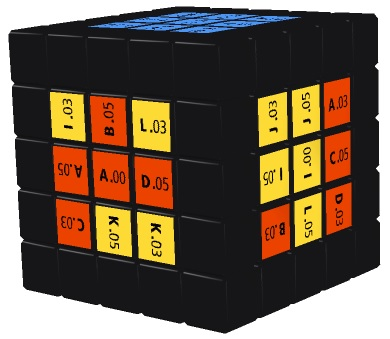Suppose we want to move the "K.03" center piece to the position occupied by "D.03" (note that these two pieces are located at corresponding positions of the front and right faces). So the positions of "K.03" and "D.03" are our X and Y, respectively. To determine Z, we need to see what position "K.03" will reach after a 90° rotation of the front face. On the one hand, after a 90° clockwise rotation, "K.03" will reach the position occupied by "C.03". But since "C.03" and "K.03" lie on the same horizontal line, the use condition of the (XYZ) formulas is violated. On the other hand, after a 90° anticlockwise rotation, "K.03" will reach the position occupied by "L.03". Since "L.03" and "K.03" do not lie on the same horizontal line, the use condition is satisfied and so the position of "L.03" is our Z. Since "K.03" and "L.03" lie on the 3rd and 1st horizontal inner layer respectively, we may substitute 3 and 1 into x and z of the Anticlockwise (XYZ) Formula (2) and obtain:

U3−1 R−1 U1−1 R U3 R−1 U1 R

After doing the above series of rotations to the Supercube shown above, the Supercube will look like the following as desired:I next introduce five formulas which can be used to swap or flip edge pieces on the top face. Here "swapping" means interchanging the positions of two edge pieces, while "flipping" means turning over some edge pieces.

One Side Swapping Formula: Two Side Swapping Formula: Lx−1 U2 Lx−1 U2 F2 Lx−1 F2 Rx U2 Rx−1 U2 Lx2 (3) F2 Lx−1 F B−1 R2 F−1 B Rx Lx F B−1 R2 F−1 B Rx−1 F2 (4) Rx2 B2 U2 Lx U2 Rx−1 U2 Rx U2 F2 Rx F2 Lx−1 B2 Rx2 (5) U−1 F R−1 U F−1 Rx−1 Lx F U−1 R F−1 U Rx Lx−1 (6) F U−1 R Fm R−1 U F−1 R U−1 Fm−1 U R−1 (7)

In the formulas above, the subcripted variable x represents a vertical inner layer (counted from the right outer surface). The following figure illustrates the effects of these five formulas (with the variable x substituted by the value 1 in formulas (3) - (6). Here the red face is the front face):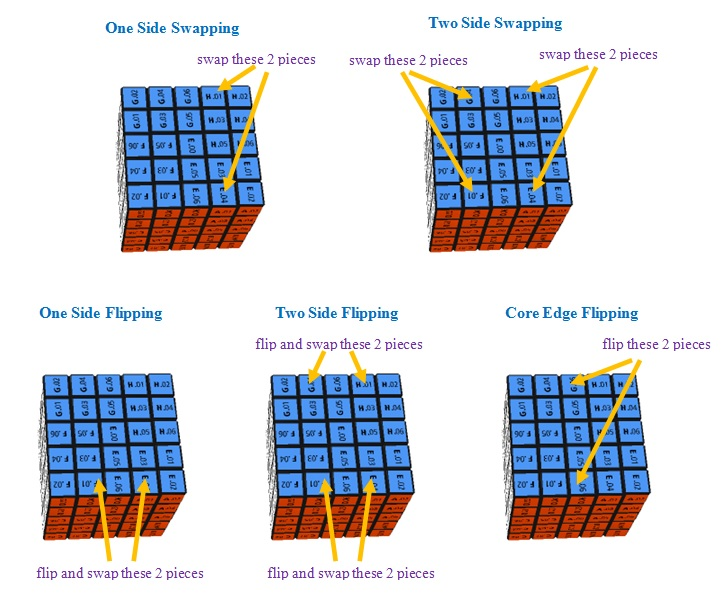By substituting different values into the variable x in formulas (3) - (6), one can swap / flip different pairs of edge pieces on the top face.

When you are playing with a Supercube with a large size, very often you need to apply the aforesaid formulas to a number of inner layers. In this case, you may have to apply the formulas several times with the variable x substituted by a different number each time. But this is tedious and error-prone. A quicker method is to treat several inner layers as one unit and move them together. For example, suppose you are playing with a 6th-order Supercube and you wish to apply Formula (3) to the 1st and 2nd inner layers counted from the right face. Instead of applying (3) twice by substituting 1 and then 2 into x of the formula, you can treat the 1st and 2nd inner layers as one unit and move them together. In this way, you only need to apply (3) once.

Note that formulas (5) and (6) do not just flip the edge pieces concerned, they also swap them. But for simplicity, I call them "Flipping Formulas". Also note that apart from swapping / flipping edge pieces as shown above, formulas (3) and (5) in fact will also move some center pieces. It is exactly this property of formual (3) that we will make use of when solving the centers of a Supercube. But for the purpose of solving the Supercube, you do not need to worry how these two formulas will affect the positions of the center pieces.

Finally, we have two formulas for rotating the centers.

 Two Center Rotation Formula: L−1 R F−1 B U−1 D Ln D−1 U B−1 F R−1 L U−n (8) One Center Rotation Formula: (U R L U2 R−1 L−1)2 (9)

In the formulas above, the variable n is an exponent and represents the number of 90° rotations of a face. The following figure illustrates the effects of these two formulas (Here the red face is the front face):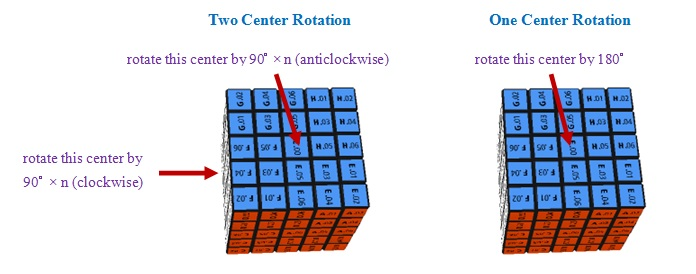Note that in the figure above, "center" refers to the whole center of a face consisting of all the center pieces, not just the core center piece.

Go to Supercube Links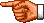Go toMy Personal Homepage (English Version)
Go toMy Personal Homepage (Chinese Version)